# Verilog 学习笔记（2）——ModelSim 的基本使用## ModelSim 的基本使用

### 如何在 ModelSim 中建立工程并完成仿真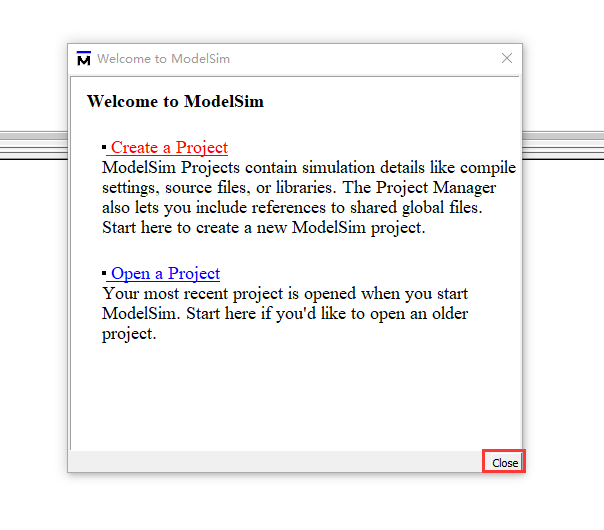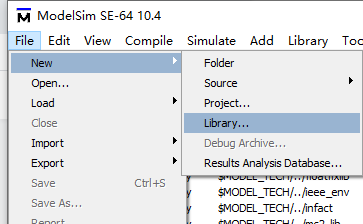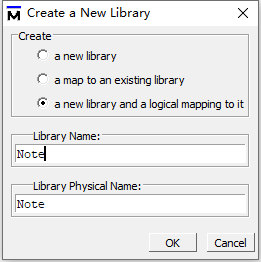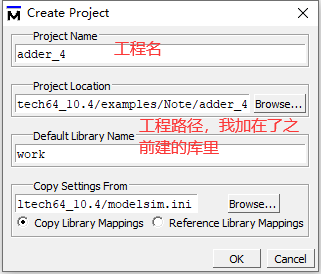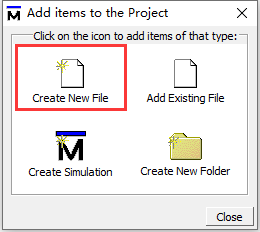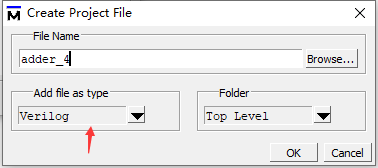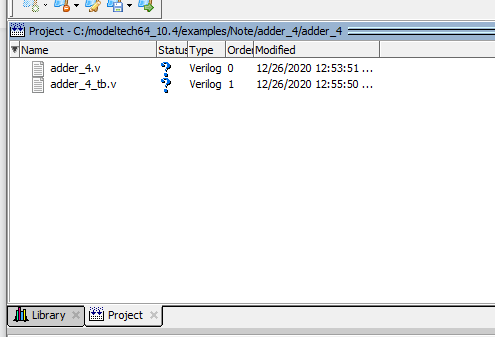proc external_editor {filename linenumber} { exec "{{VSCode路径}}\\Code.exe" -g $filename:$linenumber}


set PrefSource(altEditor) external_editor


unset PrefSource(altEditor)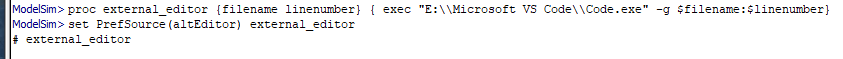module adder_4(
input [3:0] A,
input [3:0] B,
output [4:0] sum );

assign sum = A+B;

endmodule


timescale 1ns/1ns

reg  [3:0]  A;
reg  [3:0]  B;
wire [4:0]  sum;

initial begin
A = 0;
B = 0;
#10;
A = 4'b0110;
B = 4'b1010;
#10;
A = 4'd3;
B = 4'd7;
#10;
A = 5;
B = 8;
#10;
A = 4'he;
B = 4'ha;
#10;
A = 4'hf;
B = 4'hf;
end

//initial begin
//    #100 \$finish;
//end

.A(A),
.B(B),
.sum(sum)
);

endmodule
`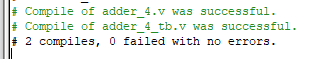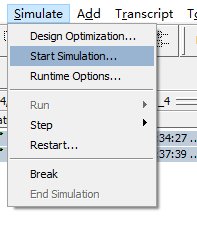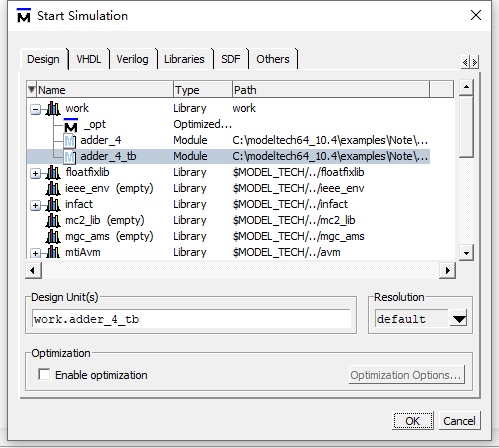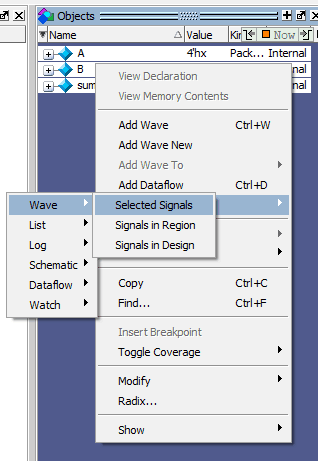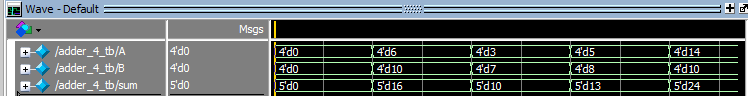### 使用 ModelSim 的一些快捷键

Modelsim 重度使用者的故事：快捷键